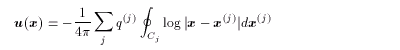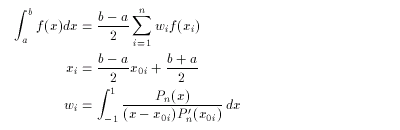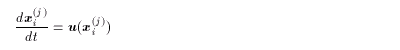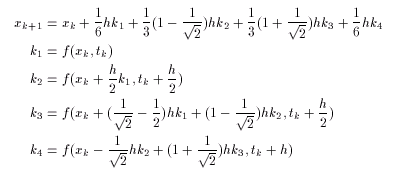3. Numerical Methods
3.1 Numerical Procedure of the Contour Dynamics Model
3.2 Other Details
3.3 Integral Calculation

### 3.3 Integral Calculation

< Velocity field >

The equation(3.12)
is calculated numerically using the Gauss-Legendre integration formula of n = 10 ( Kawakami,1989 ):(3.13)

< Time evolution >

The equation(3.14)
is integrated numerically using the Runge-Kutta-Gill scheme:(3.15)

<<index <previous next>